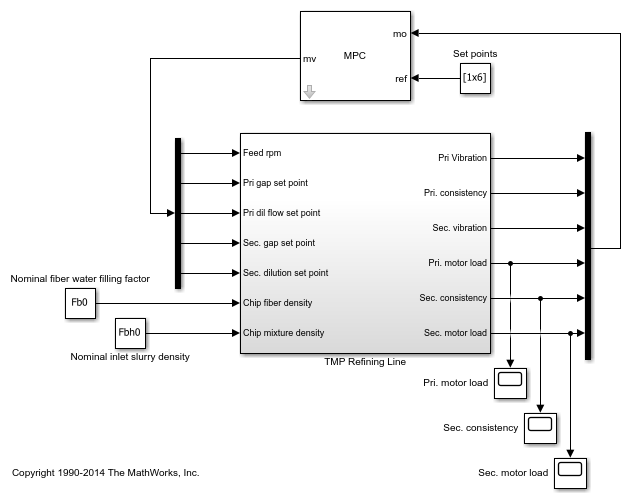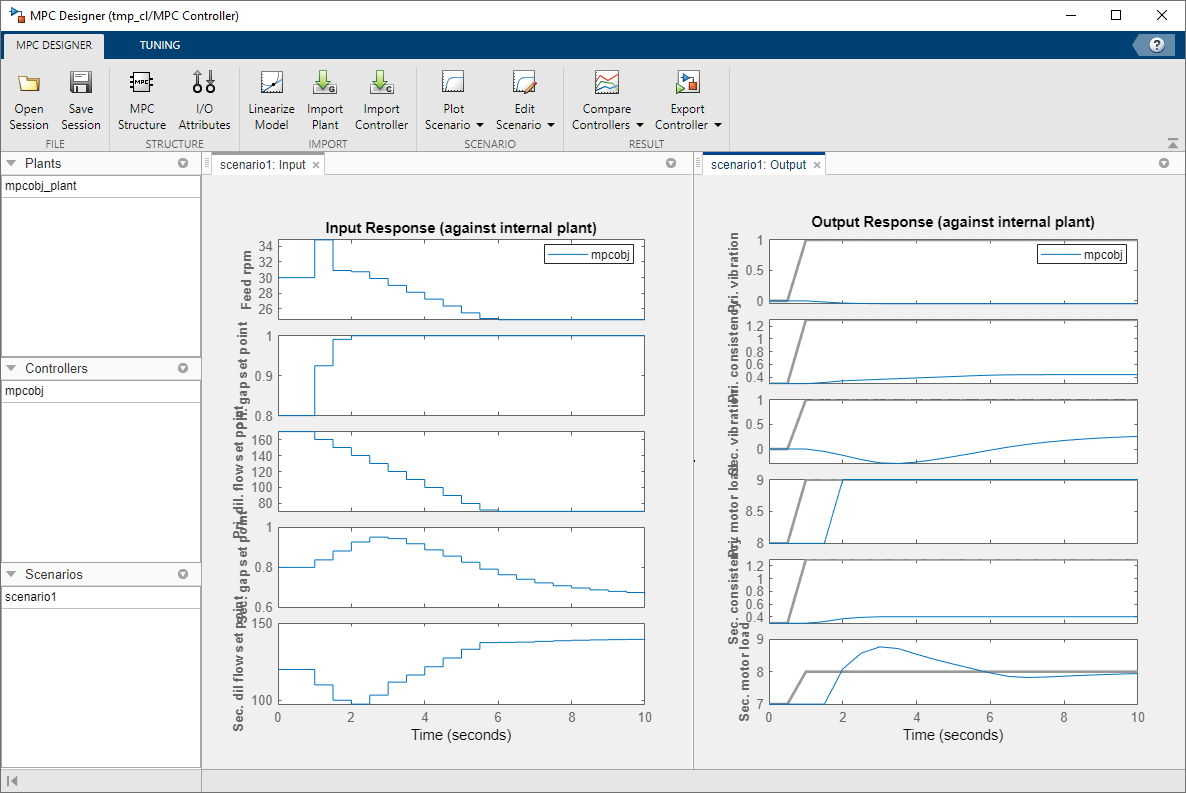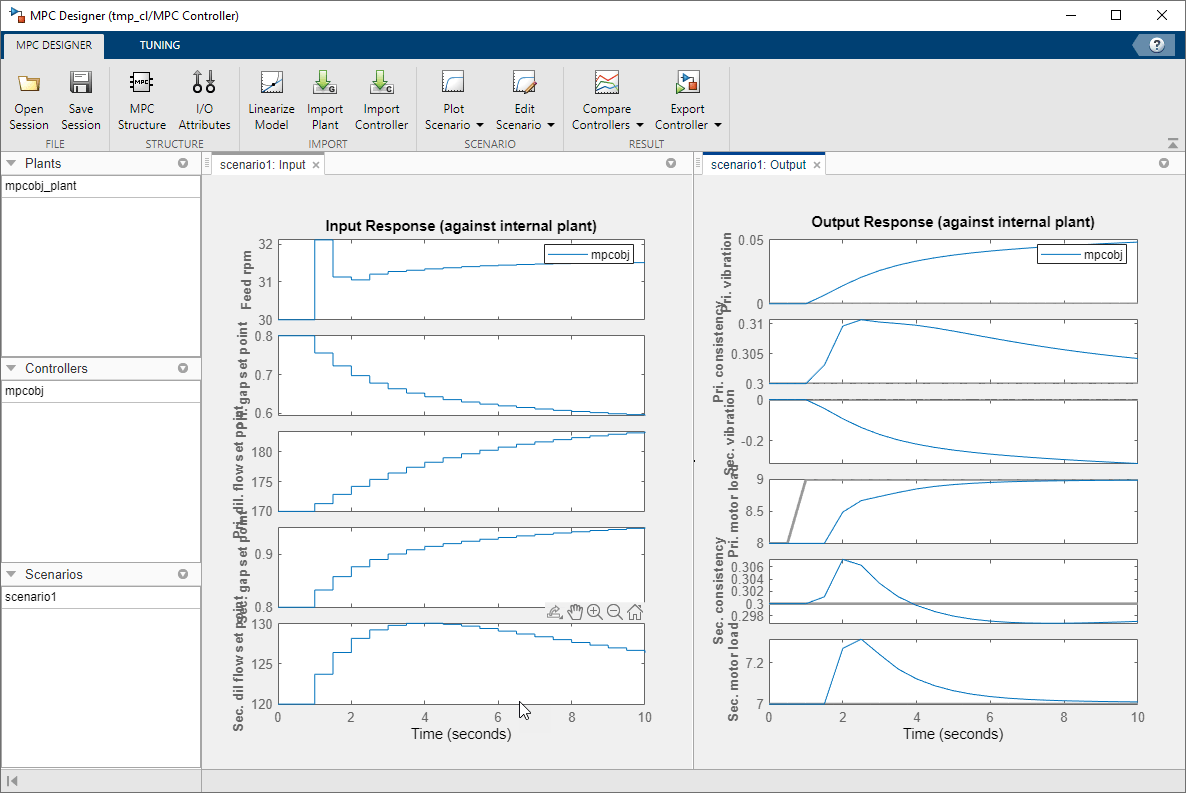Main Content

# Thermo-Mechanical Pulping Process with Multiple Control Objectives

This example shows how to control a thermo-mechanical pulping (TMP) plant with a model predictive controller.

### Plant Description

The following diagram shows a typical process arrangement for a two stage TMP operation. Two pressured refiners operate in sequence to produce a mechanical pulp suitable for making newsprint.

The primary objective of controlling the TMP plant is to regulate the energy applied to the pulp by the electric motors which drive each refiner to derive pulp with good physical properties without incurring excess energy costs.

The secondary control objective is to regulate the ratio of dry mass flow rate to overall mass flow rate (known as consistency) measured at the outlet of each refiner.

In practice, these objectives amount to regulating the primary and secondary refiner motor loads, and the primary and secondary refiner constancies, subject to the following output constraints:

(1) Maintain the power on each refiner below the maximum rated values.

(2) Maintain the vibration level on the two refiners below a critical level to prevent refiner plate clash.

(3) Limit the measured consistency to prevent blow line plugging and fiber damage.

The manipulated variables for this plant include:

• Gap controller setpoints for regulating the distance between the refiner plates

• Dilution flow rates to the two refiners

• RPM of the screw feeder

Physical limits are also imposed on each of these inputs.### Modeling of the TMP Plant in Simulink®

To run this example, Simulink® is required.

```if ~mpcchecktoolboxinstalled('simulink') disp('Simulink(R) is required to run this example.') return end ```

The following Simulink® model represents a TMP plant and an MPC Controller designed for the control objectives described above. The model is opened and plant data is initialized:

```open_system('mpc_tmpdemo') mpctmpinit; ```The MPC controller is represented by an MPC object in the workspace. It is loaded from a previously saved design:

```load mpc_tmpdemodata; mpcobj ```
``` MPC object (created on 30-Mar-2004 17:20:31): --------------------------------------------- Sampling time: 0.5 (seconds) Prediction Horizon: 20 Control Horizon: 5 Plant Model: -------------- 5 manipulated variable(s) -->| 7 states | | |--> 6 measured output(s) 0 measured disturbance(s) -->| 5 inputs | | |--> 0 unmeasured output(s) 0 unmeasured disturbance(s) -->| 6 outputs | -------------- Disturbance and Noise Models: Output disturbance model: user specified (type "getoutdist(mpcobj)" for details) Measurement noise model: user specified (type "mpcobj.Model.Noise" for details) Weights: ManipulatedVariables: [0 0 0 0 0] ManipulatedVariablesRate: [0.1000 10 0.1000 10 0.1000] OutputVariables: [0 10 0 1 10 1] ECR: 1000000 State Estimation: Default Kalman Filter (type "getEstimator(mpcobj)" for details) Constraints: 0 <= Feed rpm <= 35, -10 <= Feed rpm/rate <= Inf, -Inf <= Pri. vibration <= 1 0 <= Pri. gap set point <= 1, -10 <= Pri. gap set point/rate <= Inf, -Inf <= Pri. consistency <= 0.45 70 <= Pri. dil. flow set point <= 250, -10 <= Pri. dil. flow set point/rate <= Inf, -Inf <= Sec. vibration <= 1 ............... ............... ............... 70 <= Sec. dil flow set point <= 250, -10 <= Sec. dil flow set point/rate <= Inf, -Inf <= Sec. motor load <= 9 ```

The controller was designed using the MPC Designer app.

### Tuning the Controller Using the MPC Designer App

Click the "Design" button in the MPC Controller block dialog to launch the MPC Designer app.In the Tuning tab, click Weights to open the Weights dialog box. To put more emphasis on regulating primary and secondary refiner motor loads and constancies, specify the input and output weights as follows:In the MPC Designer tab, click Edit Scenario to open the Simulation Scenario dialog box. To simulate a primary refiner motor load setpoint change from 8 to 9 MW without a model mismatch, specify the simulation scenario settings as follows:The effect of design changes can be observed immediately in the response plots.### Simulating the Design in Simulink®

The controller can be tested on the non-linear plant by running the simulation in Simulink®. In the Tuning tab, in the Update and Simulate drop-down list, select Update Block and Run Simulation to export the current controller design to the MATLAB workspace and run the simulation in Simulink.

The output of the 3 scopes show the response to initial setpoints with:

• Primary consistency of 0.4

• Secondary motor load of 6 MW

• Secondary consistency of 0.3```bdclose('mpc_tmpdemo') ```

## Support

#### Implementing an Adaptive Cruise Controller with Simulink

Download technical paper